# How to Use 2D Transformation Functions in CSS

HTML & CSS

Share:

#### Free JavaScript Book!Write powerful, clean and maintainable JavaScript.

RRP \$11.95

The following is a short extract from Tiffany’s new book, CSS Master, 2nd Edition.

Transforms allow us to create effects and interactions that are otherwise impossible. When combined with transitions and animations, we can create elements and interfaces that rotate, dance, and zoom. Three-dimensional transforms, in particular, make it possible to mimic physical objects. In this piece, we’ll look at 2D transform functions (3D functions are covered here).

There are four primary two-dimensional transform functions: `rotate`, `scale`, `skew`, and `translate`. Six other functions let us transform an element in a single dimension: `scaleX` and `scaleY`; `skewX` and `skewY`; and `translateX` and `translateY`.

### `rotate()`

A rotation transform spins an element around its origin by the angle specified around the `transform-origin` point. Using `rotate()` tilts an element clockwise (positive angle values) or counter-clockwise (negative angle values). Its effect is much like a windmill or pinwheel, as seen below.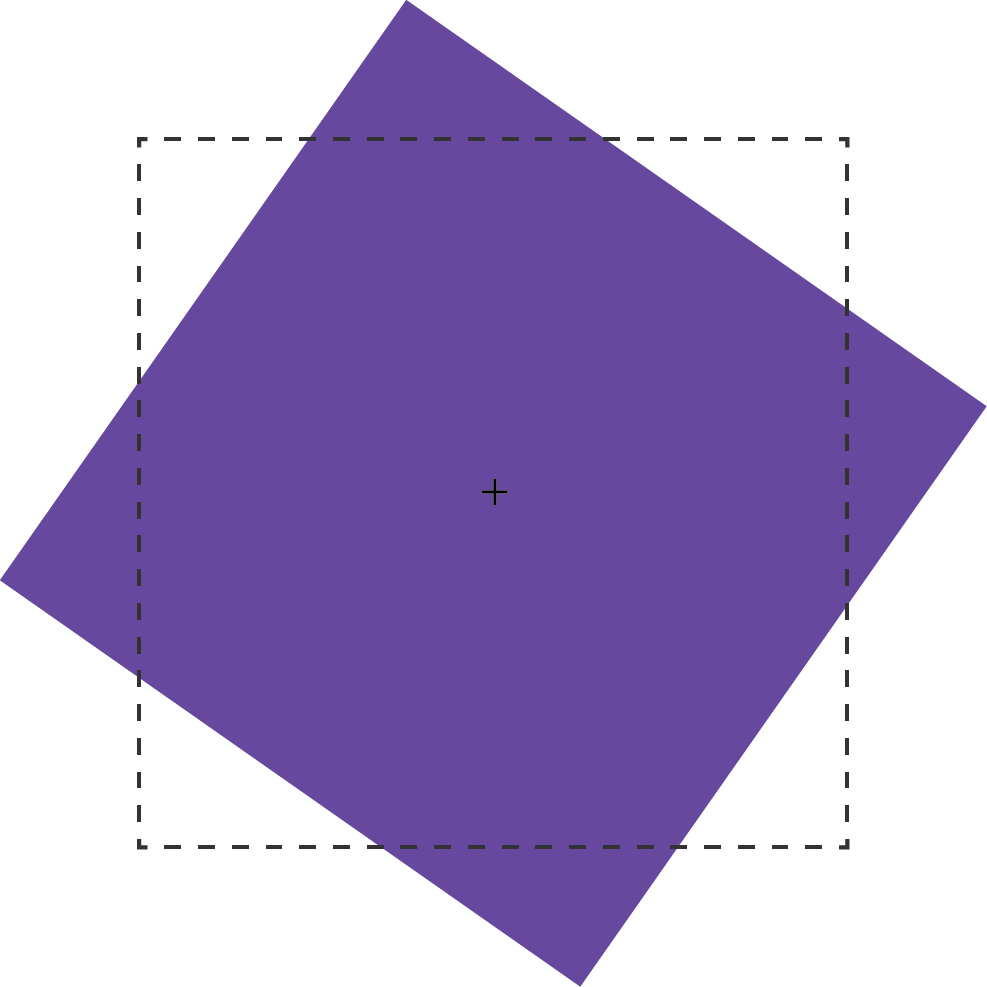The `rotate()` function accepts values in angle units. Angle units are defined by the CSS Values and Units Module Level 3. These may be `deg` (degrees), `rad` (radians), `grad` (gradians), or `turn` units. One complete rotation is equal to `360deg`, `6.28rad`, `400grad`, or `1turn`.

Rotation values that exceed one rotation (say, `540deg` or `1.5turn`) are rendered according to their remaindered value, unless animated or transitioned. In other words, `540deg` is rendered the same as `180deg` (540 degrees minus 360 degrees) and `1.5turn` is rendered the same as `.5turn` (1.5 – 1). But a transition or animation from `0deg` to `540deg` or `1turn` to `1.5turn` will rotate the element one-and-a-half times.

### 2D Scaling Functions: `scale`, `scaleX`, and `scaleY`

With scaling functions, we can increase or decrease the rendered size of an element in the X-dimension (`scaleX`), Y-dimension (`scaleY`), or both (`scale`). Scaling is illustrated below, where the border illustrates the original boundaries of the box, and the + marks its center point.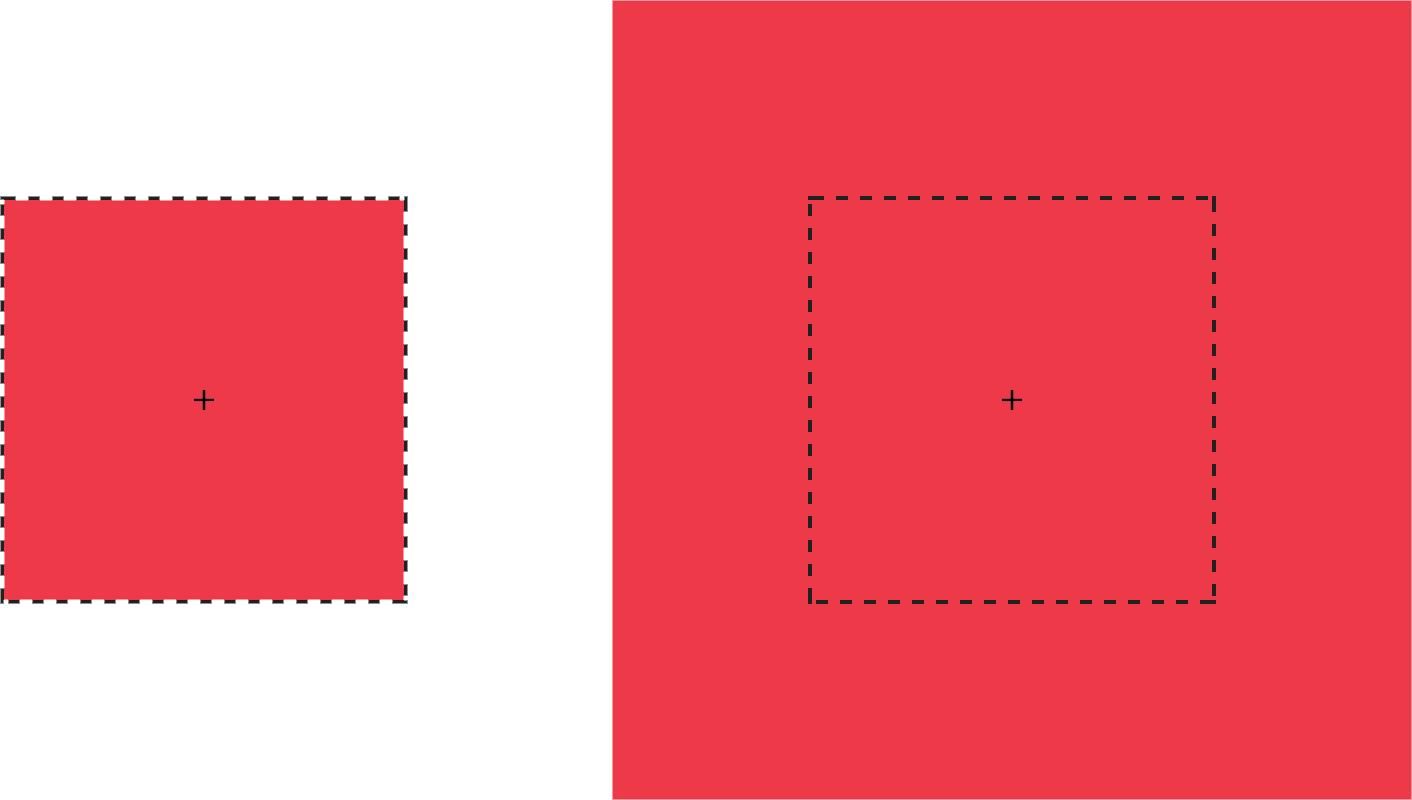Each scale function accepts a multiplier or factor as its argument. This multiplier can be just about any positive or negative number. Percentage values aren’t supported. Positive multipliers greater than `1` increase the size of an element. For example, `scale(1.5)` increases the size of the element in the X and Y directions 1.5 times. Positive multipliers between `0` and `1` will reduce the size of an element.

Values less than `0` will also cause an element to scale up or down in size and create a reflection (flip) transform.

Warning: Using `scale(0)` will cause the element to disappear, because multiplying a number by zero results in a product of zero.

Using `scale(1)` creates an identity transformation, which means it’s drawn to the screen as if no scaling transformation was applied. Using `scale(-1)` won’t change the drawn size of an element, but the negative value will cause the element to be reflected. Even though the element doesn’t appear transformed, it still triggers a new stacking context and containing block.

It’s possible to scale the X and Y dimensions separately using the `scale` function. Just pass it two arguments: `scale(1.5, 2)`. The first argument scales the X-dimension; the second scales the Y-dimension. We could, for example, reflect an object along the X-axis alone using `scale(-1, 1)`. Passing a single argument scales both dimensions by the same factor.

### 2D Translation Functions: `translateX`, `translateY`, and `translate`

Translating an element offsets its painted position from its layout position by the specified distance. As with other transforms, translating an element doesn’t change its `offsetLeft` or `offsetTop` positions. It does, however, affect where it’s visually positioned on screen.

Each 2D translation function—`translateX`, `translateY`, and `translate`—accepts lengths or percentages for arguments. Length units include pixels (`px`), `em`, `rem`, and viewport units (`vw` and `vh`).

The `translateX` function changes the horizontal rendering position of an element. If an element is positioned zero pixels from the left, `transform: transitionX(50px)` shifts its rendered position 50 pixels to the right of its start position. Similarly, `translateY` changes the vertical rendering position of an element. A transform of `transform: transitionY(50px)` offsets the element vertically by 50 pixels.

With `translate()`, we can shift an element vertically and horizontally using a single function. It accepts up to two arguments: the X translation value, and the Y translation value. The figure below shows the effect of an element with a `transform` value of `translate(120%, -50px)`, where the left green square is in the original position, and the right green square is translated 120% horizontally and -50px vertically from its containing element (the dashed border).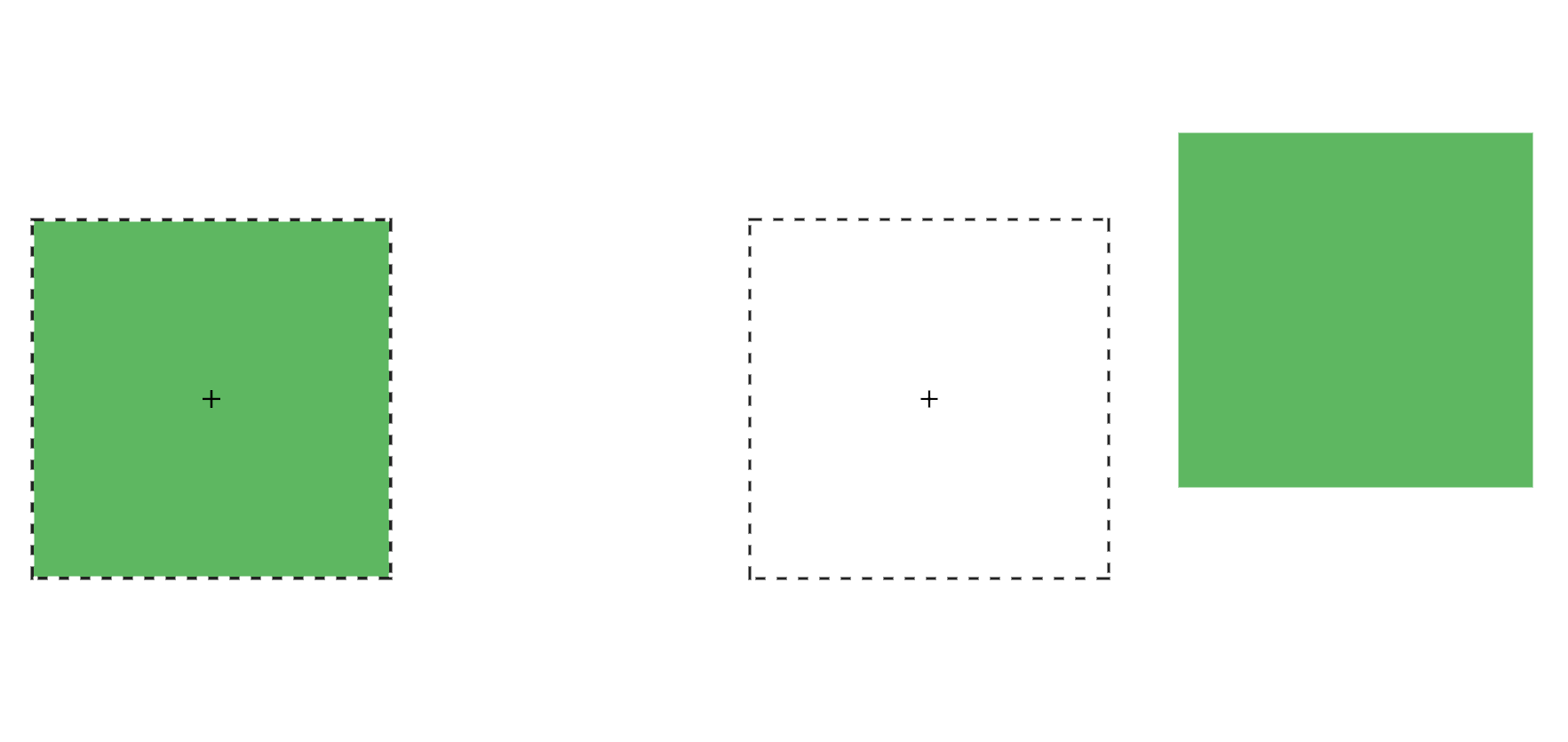Passing a single argument to `translate` is the equivalent of using `translateX`; the Y translation value will be set to `0`. Using `translate()` is the more concise option. Applying `translate(100px, 200px)` is the equivalent of `translateX(100px) translateY(200px)`.

Positive translation values move an element to the right (for `translateX`) or downward (for `translateY`). Negative values move an element to the left (`translateX`) or upward (`translateY`).

Translations are particularly great for moving items left, right, up, or down. Updating the value of the `left`, `right`, `top`, and `bottom` properties forces the browser to recalculate layout information for the entire document. But transforms are calculated after the layout has been calculated. They affect where the elements appear on screen, but not their actual dimensions. Yes, it’s weird to think about document layout and rendering as separate concepts, but in terms of browsers, they are.

#### Transform Properties May Be Coming to a Browser near You

The latest version of the CSS Transforms specification adds `translate`, `rotate`, and `scale` properties to CSS. Transform properties work much like their corresponding transform functions, but values are space-separated instead of comma-separated. We could, for example, express `transform: rotate3d(1, 1, 1, 45deg)` using the `rotate` property: `rotate: 1 1 1 45deg`. Similarly, `translate: 15% 10% 300px` is visually the same as `transform: translate3d(15%, 10%, 300px)` and `scale: 1.5 1.5 3` is the same as `transform: scale3d(1.5, 1.5, 3)`. With these properties we can manage rotation, translation or scale transformations separately from other transformations.

At the time of writing, browser support for transform properties is still pretty sparse. Chrome and Samsung Internet support them out of the box. In Firefox versions 60 and later, support is hidden behind a flag; visit `about: config` and set `layout.css.individual-transform.enabled` to `true`.

### `skew`, `skewX`, and `skewY`

Skew transformations shift the angles and distances between points while keeping them in the same plane. Skew transformations are also known as shear transformations, and they distort the shapes of elements, as seen below, where the dashed line represents the original bounding box of the element.The skew functions—`skew`, `skewX`, and `skewY`—accept most angle units as arguments. Degrees, gradians, and radians are valid angle units for the skew functions, while turn units, perhaps obviously, are not.

The `skewX` function shears an element in the X or horizontal direction (see teh image below). It accepts a single parameter, which again must be an angle unit. Positive values shift the element to the left, and negative values shift it towards the right.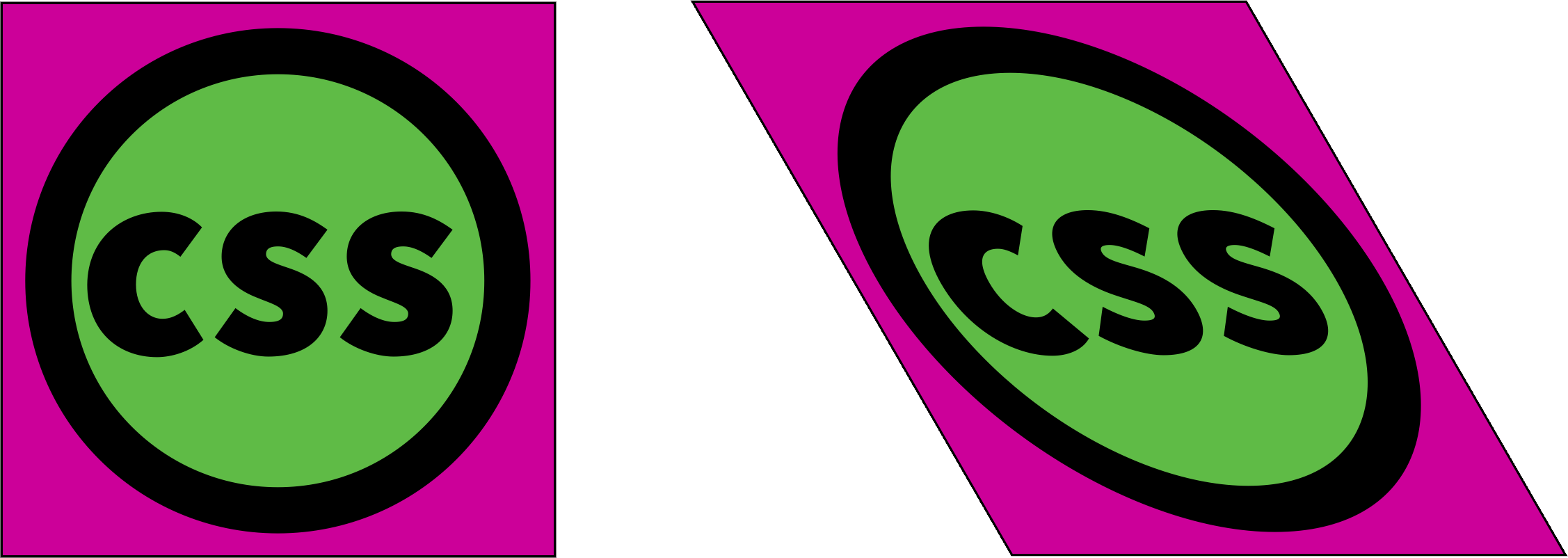Similarly, `skewY` shears an element in the Y or vertical direction. The image below shows the effect of `transform: skewY(30deg)`. Points to the right of the origin are shifted downward with positive values. Negative values shift these points upward.This brings us to the `skew` function. The `skew` function requires one argument, but accepts up to two. The first argument skews an element in the X direction, and the second skews it in the Y direction. If only one argument is provided, the second value is assumed to be zero, making it the equivalent of skewing in the X direction alone. In other words, `skew(45deg)` renders the same as `skewX(45deg)`.

## Current Transform Matrix

So far, we’ve discussed transform functions separately, but they can also be combined. Want to scale and rotate an object? No problem: use a transform list. For example:

``````.rotatescale {
transform: rotate(45deg) scale(2);
}
``````

This produces the results you see below.Order matters when using transform functions. This is a point that’s better shown than talked about, so let’s look at an example to illustrate. The following CSS skews and rotates an element:

``````.transformEl {
transform: skew(10deg, 15deg) rotate(45deg);
}
``````

It gives us the result you see below.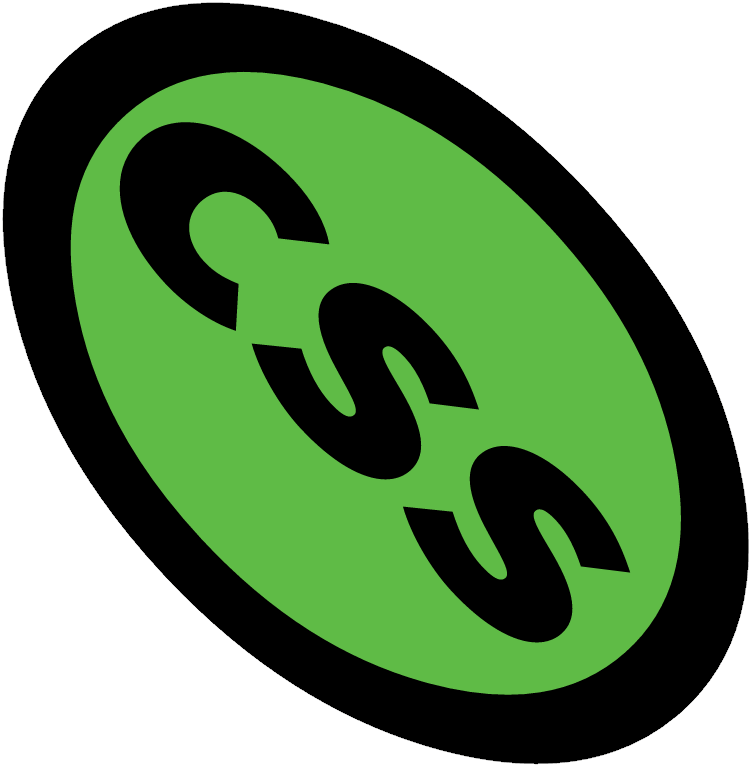What happens if you rotate an element first and then skew it?

``````.transformEl {
transform:  rotate(45deg) skew(10deg, 15deg);
}
``````

The effect, shown below, is quite different.Each of these transforms has a different current transform matrix created by the order of its transform functions. To fully understand why this is, we’ll need to learn a little bit of matrix multiplication. This will also help us understand the `matrix` and `matrix3d` functions.

## Matrix Multiplication and the Matrix Functions

A matrix is an array of numbers or expressions arranged in a rectangle of rows and columns. All transforms can be expressed using a 4×4 matrix as seen below.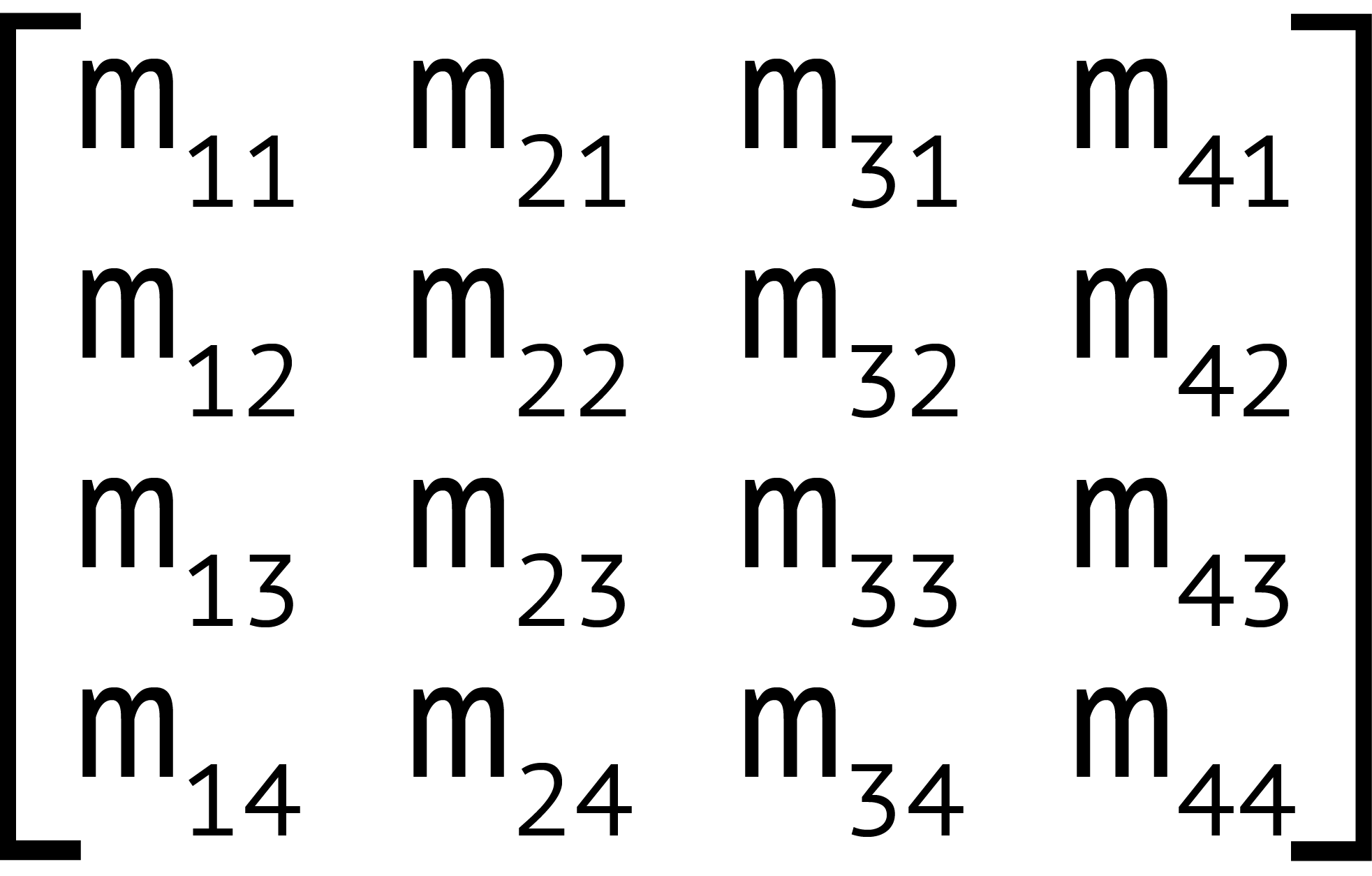This matrix corresponds to the `matrix3d` function, which accepts 16 arguments, one for each value of the 4×4 matrix. Two-dimensional transforms can also be expressed using a 3×3 matrix, seen below.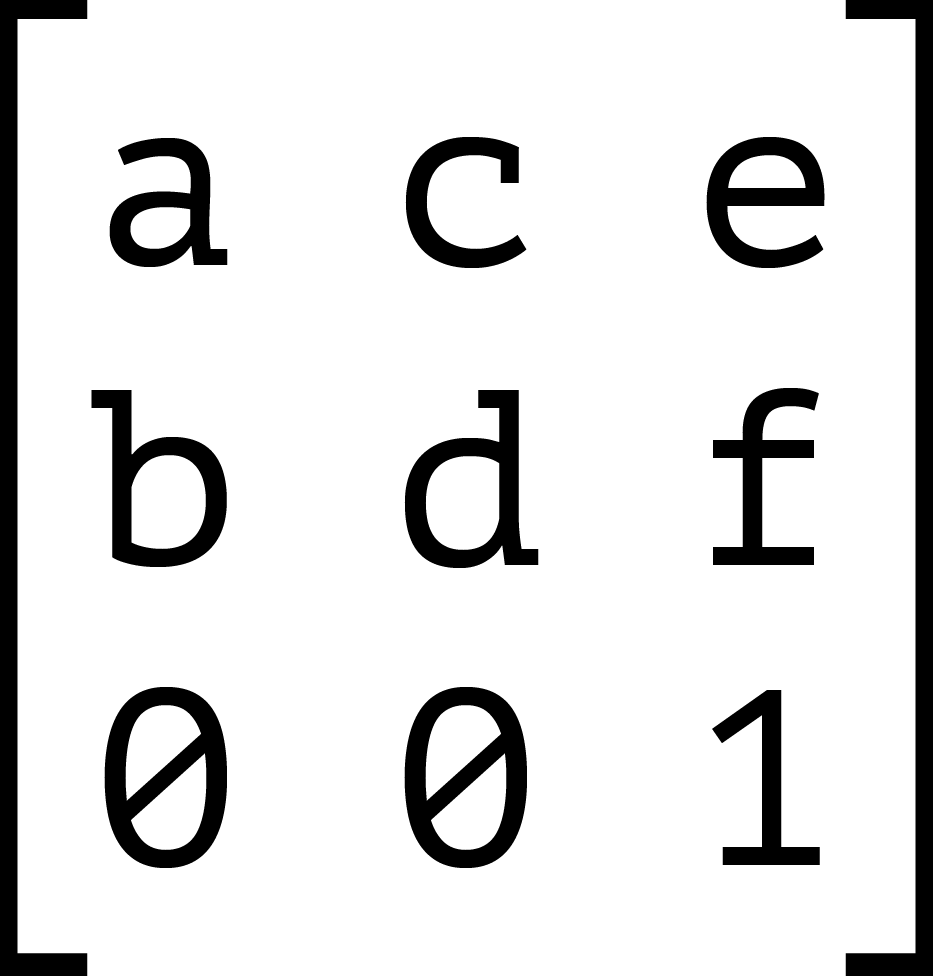This 3×3 matrix corresponds to the `matrix` transform function. The `matrix()` function accepts six parameters, one each for values a through f.

Each transform function can be described using a matrix and the `matrix` or `matrix3d` functions. The image below shows the 4×4 matrix for the `scale3d` function, where sx, sy, and sz are the scaling factors of the X, Y, and Z dimensions respectively.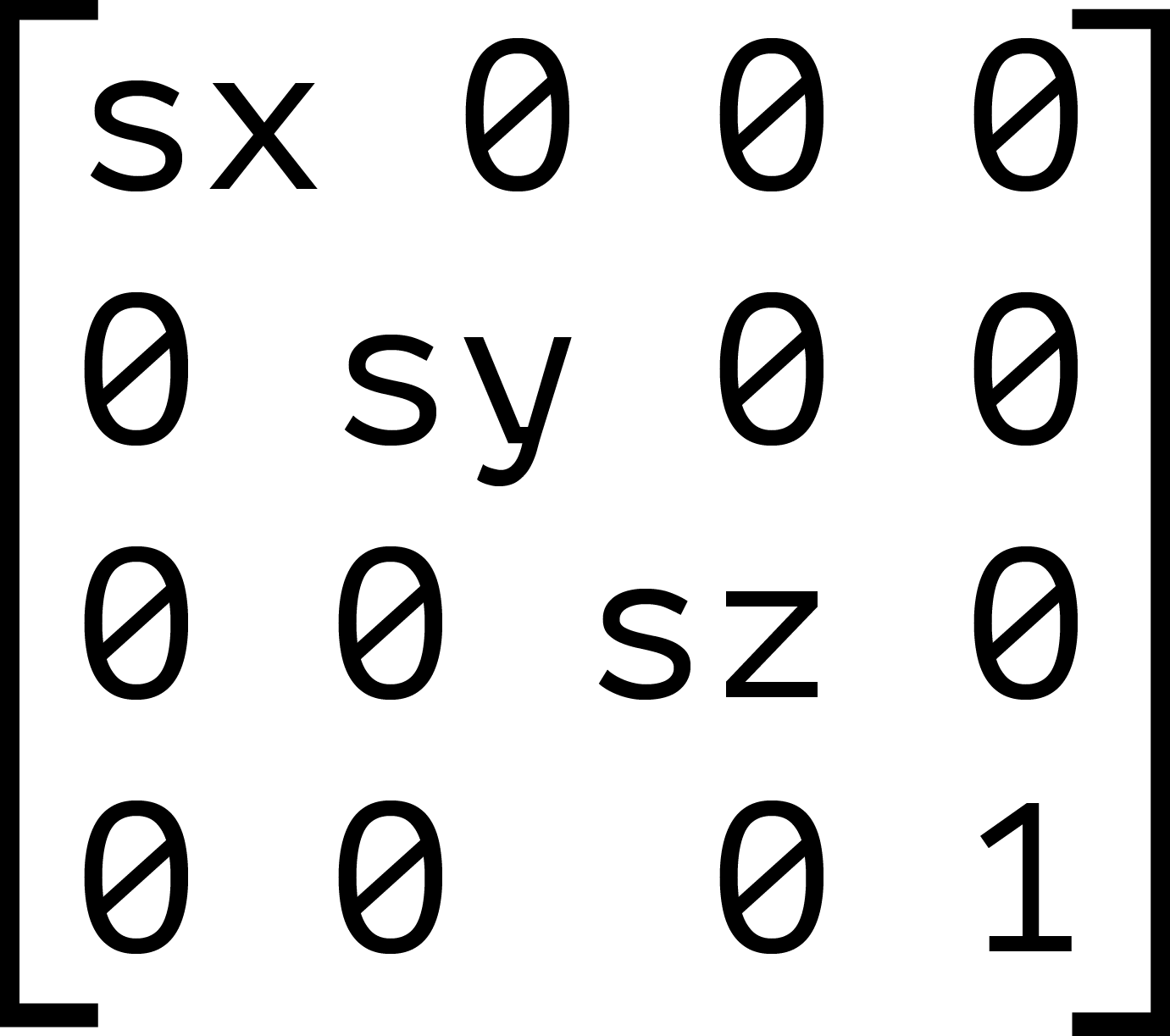When we combine transforms—such as `transform: scale(2) translate(30px, 50px)`—the browser multiplies the matrices for each function to create a new matrix. This new matrix is what’s applied to the element.

But here’s the thing about matrix multiplication: it isn’t commutative. With simple values, the product of 3×2 is the same as 2×3. With matrices, however, the product of A×B is not necessarily the same as the product of B×A. Let’s look at an example. We’ll calculate the matrix product of `transform: scale(2) translate(30px, 50px)`.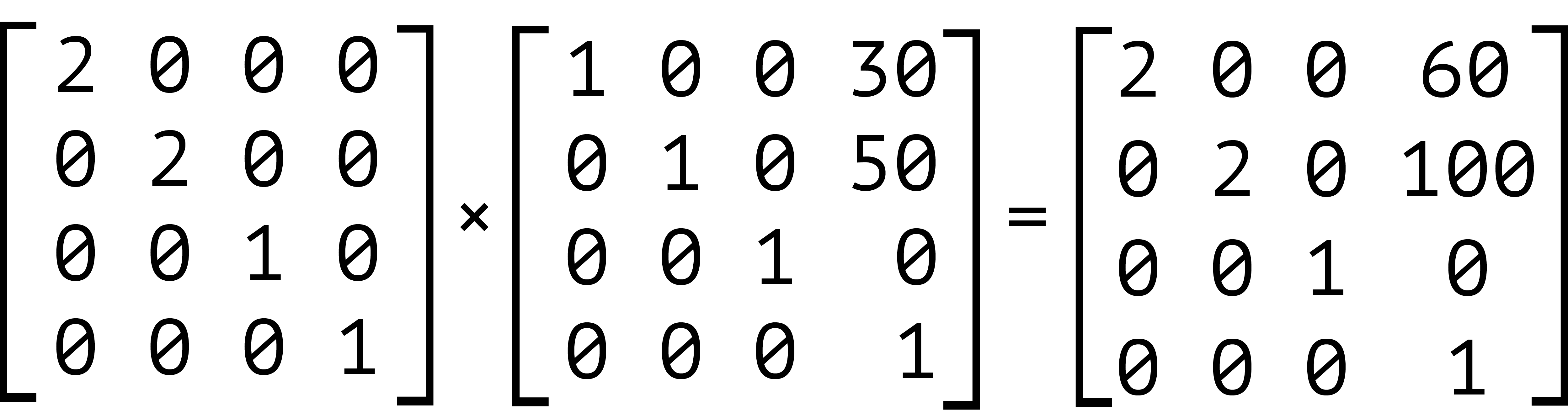Our element has been scaled by a factor of two, and then translated 60 pixels horizontally and 100 pixels vertically. We can also express this product using the `matrix` function: `transform: matrix(2, 0, 0, 2, 60, 100)`. Now let’s switch the order of these transforms—that is, `transform: translate(30px, 50px) scale(2)`. The results are shown below.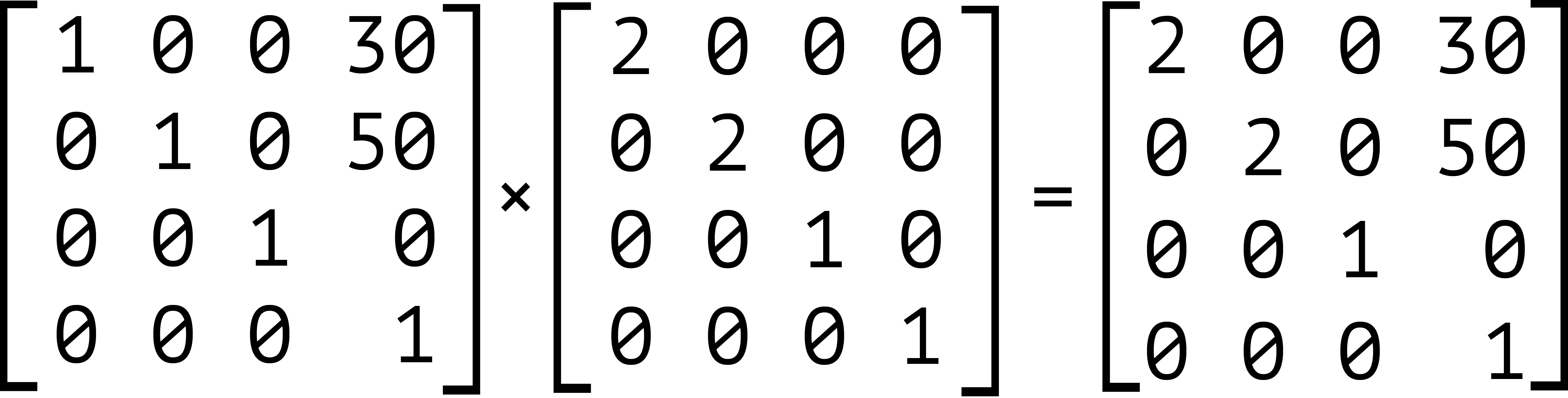Notice that our object is still scaled by a factor of two, but here it’s translated by 30 pixels horizontally and 50 pixels vertically instead. Expressed using the `matrix` function, this is `transform: matrix(2, 0, 0, 2, 30, 50)`.

It’s also worth noting that inherited transforms function similarly to transform lists. Each child transform is multiplied by any transform applied to its parent. For example, take the following code:

``````<div style="transform: skewX(25deg)">
<p style="transform: rotate(-15deg)"></p>
</div>
``````

This is rendered the same as the following:

``````<div>
<p style="transform: skewX(25deg) rotate(-15deg)"></p>
</div>
``````

The current transform matrix of the `p` element will be the same in both cases. Though we’ve focused on 2D transforms so far, the above also applies to 3D transforms. The third dimension adds the illusion of depth. It also brings some additional complexity in the form of new functions and properties.### Tiffany Brown

Tiffany B. Brown is a freelance web developer and technical writer based in Los Angeles. Brown offers web development and consulting services to larger agencies and small businesses. A former member of the Opera Software developer relations team, Brown is also co-author of SitePoint's JumpStart HTML5 book. She sporadically writes about web development technology on her blog. You can follow her on Twitter at @webinista.

#### New books out now!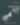Learn how Git works, and how to use it to streamline your workflow!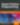Google, Netflix and ILM are Python users. Maybe you should too?